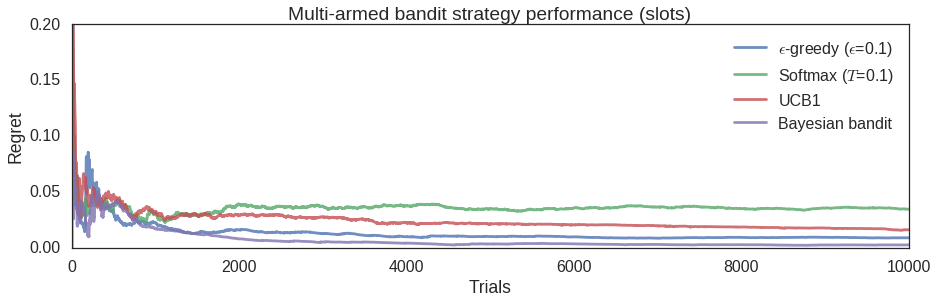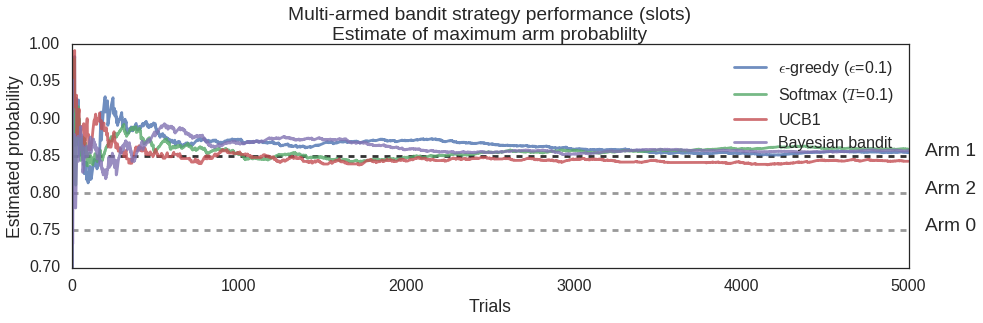Instantly share code, notes, and snippets.

# roycoding/slots.md

Last active July 1, 2022 15:29
Star You must be signed in to star a gist
slots - A multi-armed bandit library in Python

## Multi-armed banditry in Python with slots

#### Roy Keyes

22 Aug 2016 - This is a post on my blog.

I recently released slots, a Python library that implements multi-armed bandit strategies. If that sounds like something that won't put you to sleep, then please pip install slots and read on.### Multi-armed bandits

The multi-armed bandit (MAB) problem is a classic problem of trying to make the best choice, while having limited resources to gain information. The classic formulation is the gambler faced with a number of slot machines (a.k.a. one-armed bandits). How can the gambler maximize their payout while spending as little money as possible determining which are the "hot" slot machines and which are the "cold" ones? In more generic, idealized terms, you are faced with n choices, each with an associated payout probability p_i, which are unknown to you. Your goal is to run as few trials, or pulls, as possible to establish the the choice with the highest payout probability. There are many variations on this basic problem.

### Getting the best bang for your buck

Most of us do not spend our time strategizing about real slot machines, but we do see similar real-world problems, such as A/B testing or resource allocation. Because of that, strategies to solve the multi-armed bandit problem are of both practical and intellectual interest to the data scientist-types out there.

There are several strategies for solving the MAB problem. All of them attempt to strike a balance between exploration, searching for the best choice, and exploitation, using the current best choice. Because of these competing goals, determining if you are making optimal choices is not trivial. Instead of simply looking at your average payout from your trials, a quantity called regret is calculated. Intuitively, regret is the payout value lost by making the sequence of choices you have made relative to the payout you would have received having known the best choice from the start. Regret can thus be used as a stopping criterion for making a "final", best choice.

#### Example strategy: epsilon greedy

To understand how you might approach the multi-armed bandit problem, consider the simplest reasonable strategy, epsilon greedy:

• You spend the fraction e of your trials randomly trying different choices, i.e. exploration.
• For the rest of the time, 1 - e, you always choose the choice with the current highest reward rate, i.e. exploitation.

Too little time exploring the options might lead you to stay with a sub-optimal choice. Too much time spent exploring might lead you to spend unnecessary money on options that you already know are sub-optimal.

### slots

I wrote slots with two goals in mind, investigating the performance of different MAB strategies for educational purposes and creating a usable implementation of those strategies for real world scenarios. For both of these goals, a simple API with reasonable default values was desirable.

So what does slots do? Right now, as of version 0.3.0, it has implementations of a few basic MAB strategies and allows you to run those on test scenarios and with real, live data. Currently, those strategies include epsilon greedy, softmax, upper confidence bound (UCB1), and Bayesian bandits implementations.

For "real world" (online) usage, test results can be sequentially fed into an MAB object. After each result is fed into the algorithm the next recommended choice is returned, as well as whether your stopping criterion is met.

#### What slots looks like:

Using slots to determine the best of 3 variations on a live website.

mab = slots.MAB(num_bandits=3)

Make the first choice randomly, record the response, and input reward (arm 2 was chosen here). Run online_trial (input most recent result) until the test criteria is met.

mab.online_trial(bandit=2,payout=1)

The response of mab.online_trial() is a dict of the form:

{'new_trial': boolean, 'choice': int, 'best': int}

Where:

• If the criterion is met, new_trial = False.
• choice is the choice of the next arm to try.
• best is the current best estimate of the highest payout arm.

For testing and understanding MAB strategies, you can also assign probabilities and payouts to the different arms and observe the results. For example, to compare the regret value and max arm payout probability as more trials are performed with various strategies (in this case epsilon greedy, softmax, upper credibility bound (UCB1), and Bayesian bandits):

# Test multiple strategies for the same bandit probabilities
probs = [0.75, 0.85, 0.8]

strategies = [{'strategy': 'eps_greedy', 'regret': [], 'best_prob': [],
'label': '$\epsilon$-greedy ($\epsilon$=0.1)'},
{'strategy': 'softmax', 'regret': [], 'best_prob': [],
'label': 'Softmax ($T$=0.1)'},
{'strategy': 'ucb', 'regret': [], 'best_prob': [],
'label': 'UCB1'},
{'strategy': 'bayesian', 'regret': [], 'best_prob': [],
'label': 'Bayesian bandit'},
]

for s in strategies:
s['mab'] = slots.MAB(probs=probs, live=False)

# Run trials and calculate the regret after each trial
for t in range(10000):
for s in strategies:
s['mab']._run(s['strategy'])
s['regret'].append(s['mab'].regret())
s['best_prob'].append(max(s['mab'].est_payouts()))

The resulting regret evolution:The estimated payout probability of the "best" arm after each trial. In this case, the actual payout probability of the best arm is 0.85.#### Making it happen

slots is on PyPI, so you can simply install with pip install slots. Currently, slots works with both Python 2.7+ and 3.4+ and the only dependency is Numpy.

### The future

slots is open source (BSD license) and I welcome outside contributions. My desire is to make slots easy-to-use, robust, and featureful. If you are interested in slots or the multi-armed bandit problem in general, please check out the References and further reading section below.

This was a very brief overview of the rich subject of dealing with the MAB problem and the slots library. Please checkout slots and send me feedback!